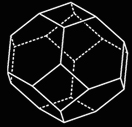# MathematicsCombinatorial objects can usually be represented as diagrams such as knots of strings, geometric polytopes or paths on a grid. Algebraic combinatorics focuses on how these objects can be used to represent formulas (say, for families of orthogonal polynomials or dimensions of graded rings) that would be difficult to compute by other means. Applications include physics, quantum information theory, knot theory and many areas of mathematics where these algebraic quantities arise.
FACULTY: Bergeron, Chan, Gao, Zabrocki,

The research interests of the analysis group at York are quite diverse, and include functional analysis and operators algebra, applied harmonic analysis, spectral theory and inverse problems, analytic number theory and the theory of the Riemann zeta function, delay differential equations and infinite dimensional dynamical systems, pseudo-differential operators and partial differential equations.
FACULTY: Farah, Gibson, SkoufranisWong, Wu, Zhu.

Category theory is a fast-growing field of pure mathematics primarily, but not exclusively, designed to detect common phenomena in distinct fields of mathematics and science, and to provide an overarching theory for them. Originating from work in algebra and topology in the 1940s, category theory has fundamentally influenced especially modern algebraic geometry, algebraic topology, logic, and significant parts of theoretical computer science.
FACULTY: Tholen

Number theory is the branch of mathematics devoted to the study of the properties of numbers in general and integers in particular. It is the one of the oldest and most fascinating areas of mathematics. Number theory is famously known for its open problems that are so accessible that they can be easily stated, yet so deep that they have withstood attempts to prove them for centuries. Notable examples include the twin prime conjecture, Goldbach’s conjecture, and the Riemann hypothesis. Research in number theory at York is centered around analytic number theory, more specifically on questions concerning the Riemann zeta function and L-functions, comparative prime number theory, character sums, and the distribution of prime numbers.
FACULTY: Ingram.

Probability theory is the mathematics of randomness, this is the mathematics that underpins statistics, actuarial science and mathematical finance, and has applications throughout physics, biology (including mathematical biology), computer science, as well as to other areas of mathematics. York’s probabilists work on a diverse set of models, including Levy processes and self-similar Markov processes, statistical physics and Monte Carlo methods, and Brownian motion and branching processes.